Share
Explore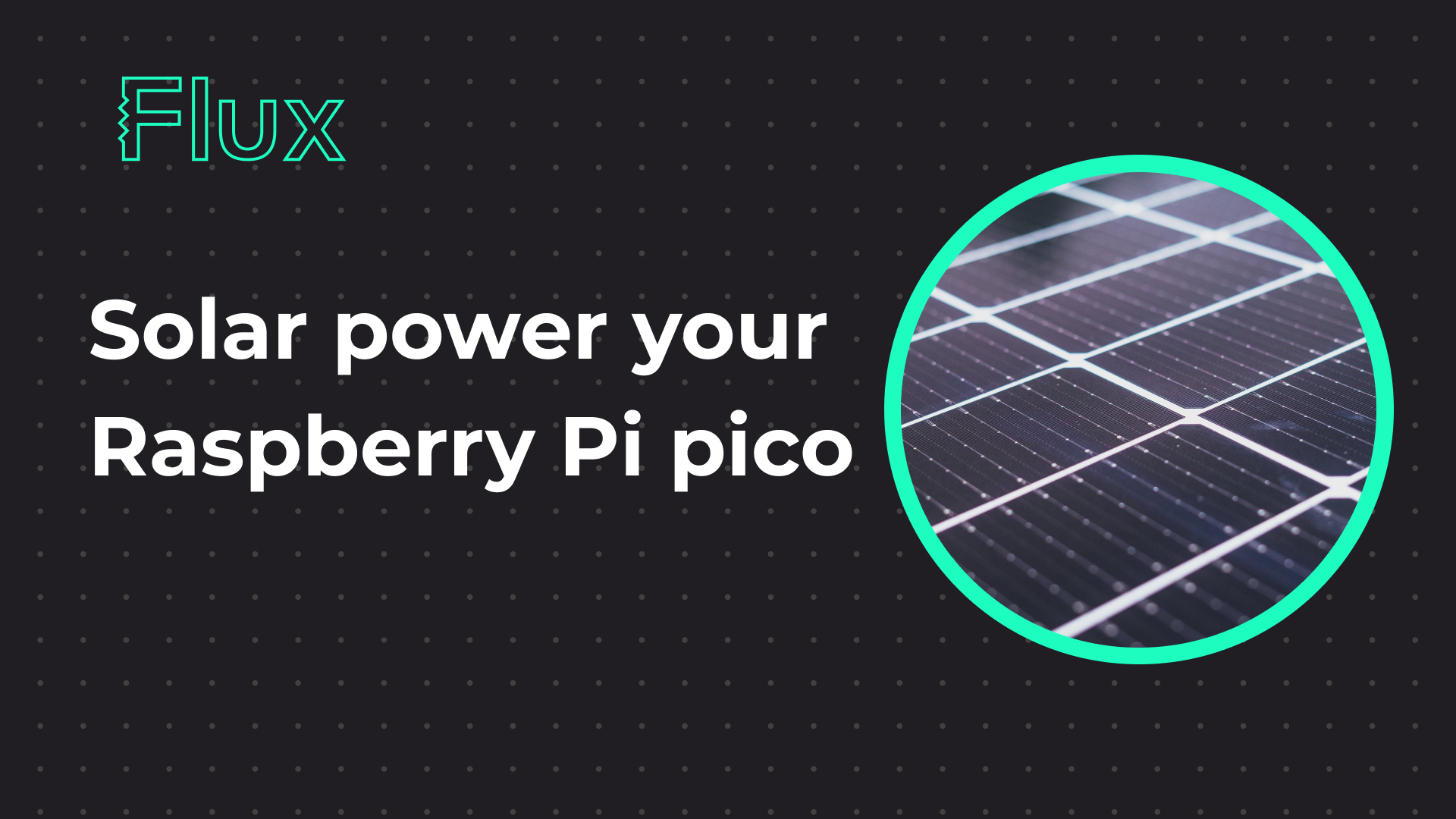## Raspberry Pi Pico power consumption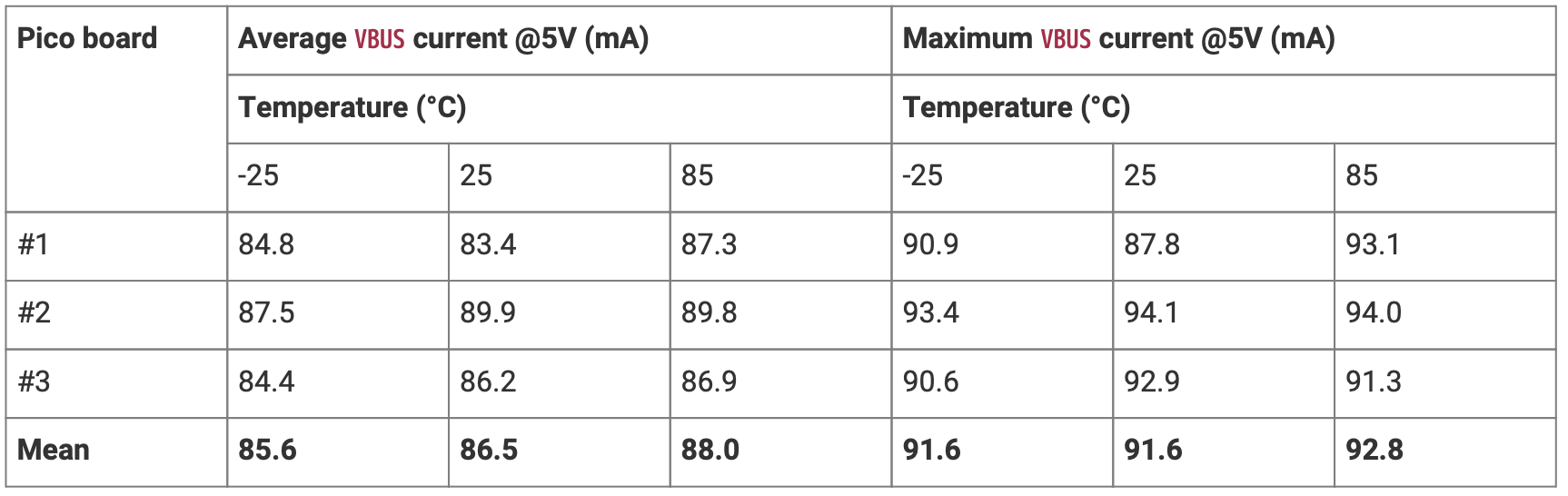Raspberry Pi pico datasheet:

From the table above, we can see that the mean maximum current required at 25C under load is 91.6mA, so we’ll need: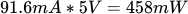That’s a pretty intense load, so let’s take half that as our reference: 230mW

## Finding the right panel size

At first glance, we could think that one should work as it can provide up to 500mW. But that’s only in perfect conditions (full sun). In order to size our panel, we need to figure out how much energy our panel will get in a day at the geographical location we intend to put it.
You can find how much solar energy your panel will receive from
As an example, we’ll take Barcelona, Spain, as the location. To make sure our panel will work even under the worst conditions, we’ll use the month of January for our calculations. From the website we get:
The average energy received per day is: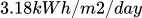In a full day, we get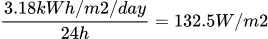The key point here is that we can’t extract 100% of the sun’s energy. From the panel datasheet, we can see that it has a surface of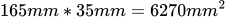, and that can it can extract 500mW. That’s only 86.5W/m2, much less than the 1000W/m2 that was shining into it.
At that efficiency (8.65%) we’re only extracting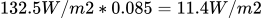To get the 230mW we need, we’d need a surface of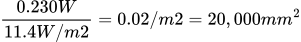, much larger than the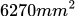of our panel.

## How does a panel work

We provided an in-depth explanation during the .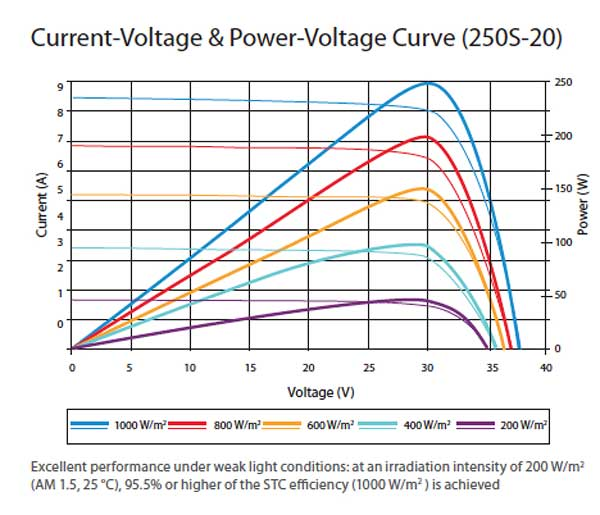## Designing a charging circuit

Circuit

Panel

Chip

### Sizing the supercapacitor

To see how much our circuit will last with the supercapacitors, we need 3 values:
The current consumed by our circuit: I=230mW@3.3V=70mA
The max voltage that the circuit charges the capacitor: Vmax=4.5V
The min voltage that the circuit charges the capacitor: Vmin=3.6V
The value of the supercapacitor: C=1F

Time =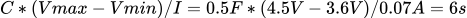Share